LINEAR ALGEBRA (Math 211)
HW #11 (Due Mon. Nov. 6 )

1. Determine which sets are vector spaces under the given operations. For those which are not, explain why.

(a) the set of triangular matrices with the usual addition and scalar multiplication;

(b) the set of matrices having the form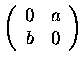with the usual addition and scalar multiplication;

(c) the set of polynomials of degree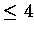( ax4+bx3+cx2+dx+e) with the usual operations;

(d) the set of polynomials of degreewith the highest coefficient 1 ( x4+ax3+bx2+cx+d) and
the usual operations;

(e) the set of all pairs of real numbers (x,y) with the operations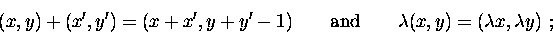(f) the set of all pairs of positive real numbers (x,y) with the operations2. Determine whether or not the following vectors span.

 ( a)    (1,2) and (-1,1) ( c)    (-2,1), (1,3) and (2,4) ( b)    (2,3) and (4,6) ( d)    (-1,2), (1,-2) and (2,-4)

3. Which of the following polynomials span the vector space of polynomials of degree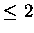(ax2+bx+c)

 ( a)    1, x2, x2-2 ( c)    x+2, x+1, x2-1 ( b)    2, x2, x, 2x+3 ( d)    x+2, x2-1

4. Letand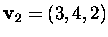. Determine whether or not the following vector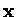belongs to(if yes" representas a linear combination of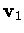and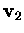)

 ( a)( b)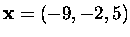.

5. Let U and V be subspaces of a vector space W. Prove thatis also a subspace of W.